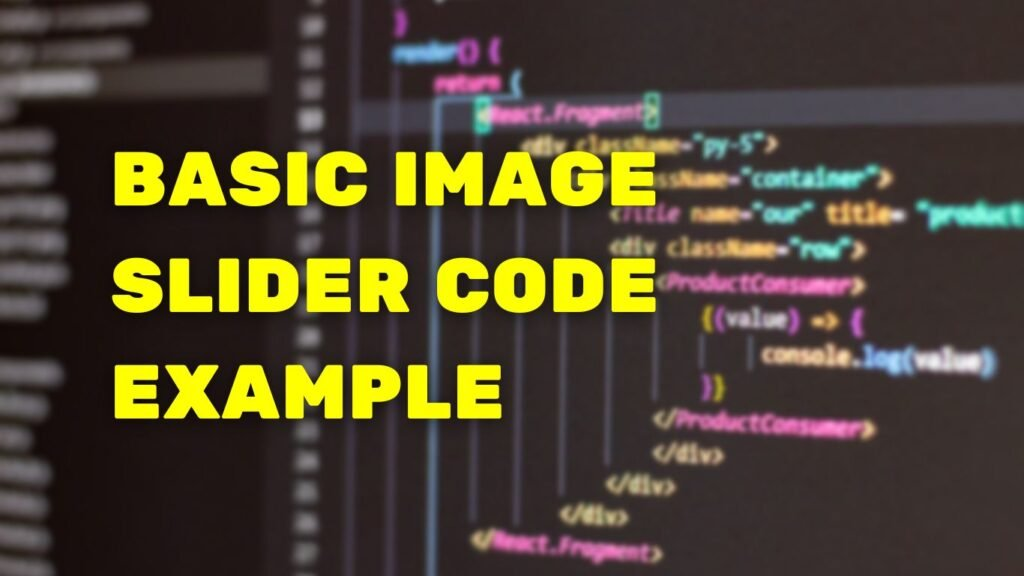# Basic Image Slider Code ExampleHere’s an example of a basic image slider using HTML, JavaScript and CSS.

## HTML

The HTML creates a container for the slider and individual slide elements for each image.

```				```
<div class="slider">
<div class="slide"><img decoding="async" src="image1.jpg"></div>
<div class="slide"><img decoding="async" src="image2.jpg"></div>
<div class="slide"><img decoding="async" src="image3.jpg"></div>
</div>

```
```

## JAVASCRIPT

The JavaScript controls the functionality of the slider, including advancing through the slides, displaying the current slide, and hiding the other slides.

```				```
var slideIndex = 1;
showSlides(slideIndex);

function plusSlides(n) {
showSlides(slideIndex += n);
}

function currentSlide(n) {
showSlides(slideIndex = n);
}

function showSlides(n) {
var i;
var slides = document.getElementsByClassName("slide");
if (n > slides.length) {slideIndex = 1}
if (n < 1) {slideIndex = slides.length}
for (i = 0; i < slides.length; i++) {
slides[i].style.display = "none";
}
slides[slideIndex-1].style.display = "block";
}

```
```

## CSS

The CSS styles the container and the slide elements so that they fill the available space and center the images.

```				```
.slider {
width: 100%;
overflow: hidden;
}

.slide {
width: 100%;
display: flex;
align-items: center;
justify-content: center;
}

.slide img {
width: 100%;
}

```
```

This code uses a combination of HTML, CSS, and JavaScript to create a basic image slider.

Please note that this is a basic example, you can use this as a starting point and add more functionality to it as per your requirement

END
Scroll to Top